# Mirrors and LensesPage 1

#### WATCH ALL SLIDES

Slide 1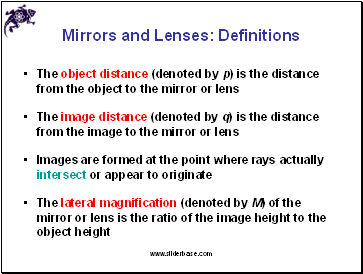## Mirrors and Lenses: Definitions

The object distance (denoted by p) is the distance from the object to the mirror or lens

The image distance (denoted by q) is the distance from the image to the mirror or lens

Images are formed at the point where rays actually intersect or appear to originate

The lateral magnification (denoted by M) of the mirror or lens is the ratio of the image height to the object height

Slide 2## Types of Images for Mirrors and Lenses

A real image is one in which light actually passes through the image point

Real images can be displayed on screens

A virtual image is one in which the light does not pass through the image point

The light appears to diverge from that point

Virtual images cannot be displayed on screens

To find where an image is formed, it is always necessary to follow at least two rays of light as they reflect from the mirror

Slide 3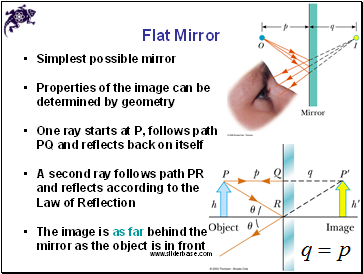## Flat Mirror

Simplest possible mirror

Properties of the image can be determined by geometry

One ray starts at P, follows path PQ and reflects back on itself

A second ray follows path PR and reflects according to the Law of Reflection

The image is as far behind the mirror as the object is in front

Slide 4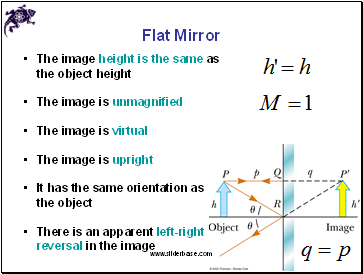Flat Mirror

The image height is the same as the object height

The image is unmagnified

The image is virtual

The image is upright

It has the same orientation as the object

There is an apparent left-right reversal in the image

Slide 5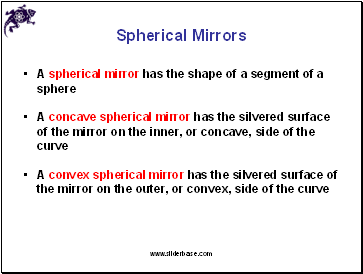## Spherical Mirrors

A spherical mirror has the shape of a segment of a sphere

A concave spherical mirror has the silvered surface of the mirror on the inner, or concave, side of the curve

A convex spherical mirror has the silvered surface of the mirror on the outer, or convex, side of the curve

Slide 6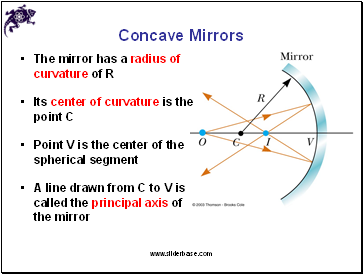## Concave Mirrors

The mirror has a radius of curvature of R

Its center of curvature is the point C

Point V is the center of the spherical segment

A line drawn from C to V is called the principal axis of the mirror

Slide 7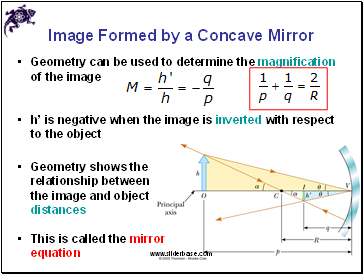## Image Formed by a Concave Mirror

Geometry can be used to determine the magnification of the image

h’ is negative when the image is inverted with respect to the object

Geometry shows the relationship between the image and object distances

Go to page:
1  2  3  4  5  6  7